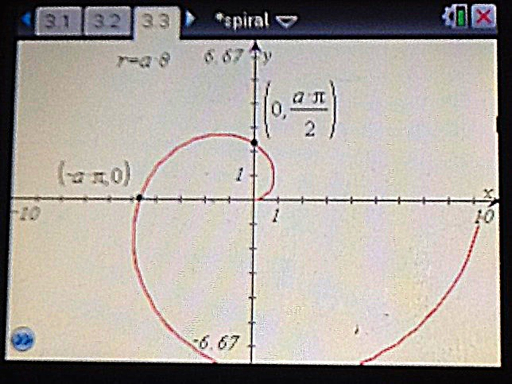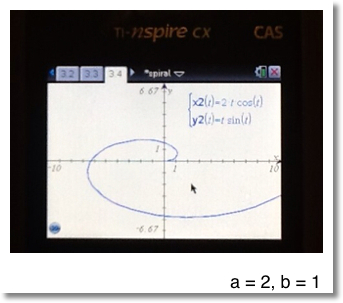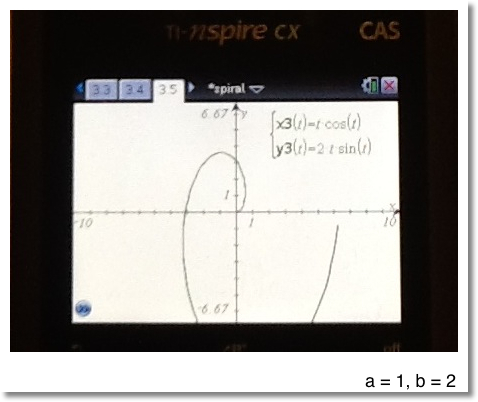## Tuesday, March 6, 2012

### Spirals

This blog will about spirals. Why? I thought it would be fun. And I like spirals.

Regular Spirals

Source: http://www.mathematische-basteleien.de/spiral.htm

Equations:
Rectangular
x^2 + y^2 = a^2 (arctan y/x)^2

Polar
r = a θ

Parametric
x = a t cos t
y = a t sin tFinding the y-intercept and x-intercept, as shown above, of a spiral, assuming 0 ≤ θ ≤ 2 π

We can use the rectangular equation to find these points.

x^2 + y^2 = a^2 (arctan y/x)^2

Y-Intercept - Point (0, y)

y^2 = a^2 (arctan y/0)^2

Note:
1. y/0 is undefined
2. tan (π /2) is undefined. So is tan (n π / 2) where n is an integer.

Use π / 2 as a solution.

Then:

y^2 = a^2 * (π / 2)^2

Take the square root of both sides, since y>0, use the positive root.

y = a * π / 2

The y-intercept is (0, a * π / 2)

X-Intercept - Point (x, 0)

x^2 = a^2 (arctan 0)^2

Note tan(n π.)=0 where n is an integer. Let's use π. (n = 1)

x^2 = a^2 π^2

Since the x-intercept lies left of 0, select the negative square roof. Therefore:

x = -a π.

The x-intercept is (-a π, 0).

Area of a Spiral (0 ≤ θ ≤ 2 π)

Use the polar equation: r = a θ

Using the general polar integral:

A = ∫ (1/2 * r^2 d θ , lower limit, upper limit)

A = ∫ (1/2 * a^2 * θ^2 d θ , 0, 2 π)
= a^2 / 2 * (8 π^3 / 3 - 0)
= 4 a^2 π^3 / 3

Slope of a Spiral

Polar Form:

∂r/∂θ = a

Parametric Form:

∂x/∂t = ∂/∂t (a * t * cos t)
= ∂/∂t (a * t) * cos t + a * t * ∂/∂t (cos t)
= a * cos t - a * t * sin t
= a * (cos t - t * sin t)

∂y/∂t = ∂/∂t (a * t * sin t)
= ∂/∂t (a * t) * sin t + a * t * ∂/∂t (sin t)
= a * sin t + a * t * cos t
= a * (sin t + t * cos t)

Stretch Spirals

What if we can "stretch" spirals? We can if alter the parameters of the parametric form:

x(t) = a * t * cos t
y(t) = b * t * sin t

Where a ≠ b.

Two graphical examples are shown below.Finding the Intercepts of a Stretched Spiral

I will assume that both a ≠ 0 and b ≠ 0.

Y-Intercept: Point (0, y)

0 = a * t * cos t
y = b * t * sin t

Working with the first equation:

0 = a * t * cos t
0 = t * cos t,

which implies that either t = 0 or cos t = 0

We know that cos (n π / 2) = 0 where n is an integer. Choose t = π / 2.

Then y = b * π / 2 * sin(π / 2) = b * π / 2

Then the y-intercept is (0, π / 2).

X-Intercept: Point (x, 0)

x = a * t * cos t
0 = b * t * sin t

Working with the second equation:
0 = b * t * sin t
0 = t * sin t

which implies that t = 0 or sin t = 0. We know that sin(n π) = 0 where n is an integer, let's choose n = 1 and then t = π.

Hence x = a * π * cos π = -a * π.

The x-intercept is (-a * π, 0)

Area of a Stretched Spiral

We can find the area using the following:

∫ y dx = ∫ y(t) d[x(t)]

Note that:
1. y(t) = b * t * sin t
2. d[x(t)] = a * (cos t - t * sin t) dt
3. y(t) d[x(t)] = a * b * (t * sin t * cos t - t^2 * sin^2 t)

Let Φ = (t * sin t * cos t - t^2 * sin^2 t)

The integration is split into two intervals: [0, π] and [π, 2 π]. Due to the direction of the spiral, the limits are switched.

Then the area is:
A = a * b * ( ∫ (Φ dt, π , 0 ) + ∫ ( Φ dt, 2 π , π ) )
(via Hewlett Packard HP 50g and TI nSpire CX CAS)
= a * b * (π^3 / 6 + 7 * π^3 / 6)
= a * b * (4 π / 3)

Slope of a Stretched Spiral

The derivation is similar to the slope of a regular spiral.

∂x / ∂t = a * ( cos t - t * sin t )
∂y / ∂t = b * ( sin t + t * cos t )

Until next time, Eddie.

This blog is property of Edward Shore. (c) 2012

### Casio fx-CG50: Sparse Matrix Builder

Casio fx-CG50: Sparse Matrix Builder Introduction The programs can create a sparse matrix, a matrix where most of the entries have zero valu...